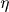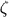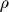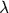### Derek M. Johnson and Carol C. Horvitz. 2005. Estimating postnatal dispersal: tracking the unseen dispersers. Ecology 86:1185–1190.

Appendix C. Sensitivity analyses: the effects of variation in model parameters and model assumptions on recolonization rate in the flood zone.

Parameter values for,em,,im, SE, Sp, SA,nf, and SA,f were changed to their upper and lower 95% percent confidence limits, one parameter at a time, and the model simulation was rerun to estimate the sensitivity of beetle population dynamics in the flood zone to variation in the parameters and its effects on estimation of the post-natal dispersal parameter.  We do not have a good measure of the 95% confidence interval for larval survival probability (SL) during non-flood events, thus, we tested the sensitivity of the model and its predictions to a wide range of probabilities (0.5–1.0).  Also, SE,f and SP,f were changed to SE,nf and SP,nf (non-flood probabilities) respectively, to evaluate the sensitivity of beetle population dynamics in the flood zone to flooding having no effect on survivorship of eggs and pupae.  Larval survival was not varied because there is strong evidence that flooding kills virtually 100% of larvae (Johnson 2004).  We also tested for the sensitivity of the model to the assumption that population growth of C. fenestrata is equal to one.  In a previous study, Johnson (2004) estimated population growth rate of C. fenestrata to be 1.02 ± 0.06 SE. We varied the population growth rate by two standard errors around the estimated level by varying the reproduction parameter (R). We also tested for the potential effect of remote migration from outside of the study area on recolonization of the flood zone. We used an estimate of remote migration from a previous study (Johnson 2003). See table for sensitivity of post-natal dispersal estimates to variations in these parameters. In this study, we made the assumption that post-natal dispersal probability was independent of natal patch size. We reran the analysis assuming that post-natal dispersal was equally dependent on patch size as was subsequent dispersal (soem = -0.51 andreplacedin Eq. 3) and found that at the maximum realistic dispersal probability (= 1) the model still underestimated recolonization of the flood zone following a flood by 34%.  Lastly, in this study we assumed that the dispersal kernel for C. fenestata was a power decay function because in a concurrent study we found that this function best described regional dynamics of the beetle (Johnson 2003).  To test the sensitivity of the results to this assumption, we reran the analysis using an exponential decay function (e-dwhere= 0.02 was estimated from the mark-recapture data using the same methods as were the parameters for the power decay function).  The model with exponential decay predicted a lower probability of post-natal dispersal (= 0.49 compared to 0.81) than the model with the power decay function, and was an approximately equally good fit to the recolonization data.  Thus, the estimate of post-natal dispersal probability was most sensitive to the assumption of power decay dispersal kernel, and was secondarily sensitive to 95% CI changes in the dispersal parameter, which predicted a range of post-natal dispersal probability () from 0.58 to 1.00. Thus, although there is a wide confidence interval for post-natal dispersal, these results demonstrate that it is still likely much greater than subsequent dispersal probabilities (= 0.09 per 25 d assuming that population size is proportional to patch size, which is supported by the data, Johnson ).

Parameter

Estimate and [95% confidence interval]

Underestimate of recolonization of the flood zone

Estimates of post-natal dispersal probability () with 95% CI variation in the given parameter

####0.78 [0.57, 1.00]

84% – 90%

0.76 – 0.86em

-0.51 [-0.58, -0.45]

82% – 91%

0.76 – 0.852.05 [1.61, 2.55]

83% – 91%

0.58 – 1.00im

0.57 [0.40, 0.88]

86% – 88%

0.80 – 0.81

SE

0.18 [0.12, 0.24]

85% – 88%

0.79 – 0.83

SL

0.87 [0.50, 1.00]

77% – 89%

0.78 – 1.00

SP

0.68 [0.59, 0.77]

86% – 87%

0.80 – 0.81

SA,nf

0.73 [0.55, 0.91]

80% – 92%

0.74 – 0.96

SA,f

0.09 [0.00, 0.25]

86% – 87%

0.76 – 0.831.02 [0.90, 1.14]§

82% – 90%%

0.77 – 0.91

M

2.46 (per 6 mo)||

86%

0.80

† Column 3 is the simulation model's percentage underestimate of the number of recolonizers of the flood zone following a flood compared to the observed number of recolonizers.

‡ The range of parameter values for larval survival probability was set above what we considered to be reasonable estimates.

§ Population growth rate was varied to 2 SE from the mean by varying the reproductive parameter R between 1.3 and 13.

|| Remote migration (m), immigration from outside of the study area was estimated based on a previous study (Johnson 2003).

LITERATURE CITED

Johnson, D. M. 2003. Spatial analyses of a Neotropical beetle: Cephaloleia fenestrata (Hispinae: Chrysomelidae: Coleoptera). Dissertation. University of Miami, Coral Gables, Florida, USA.

Johnson, D. M. 2004. Source-sink dynamics in a temporally heterogeneous environment. Ecology 85:2037–2045.

[Back to E086-065]